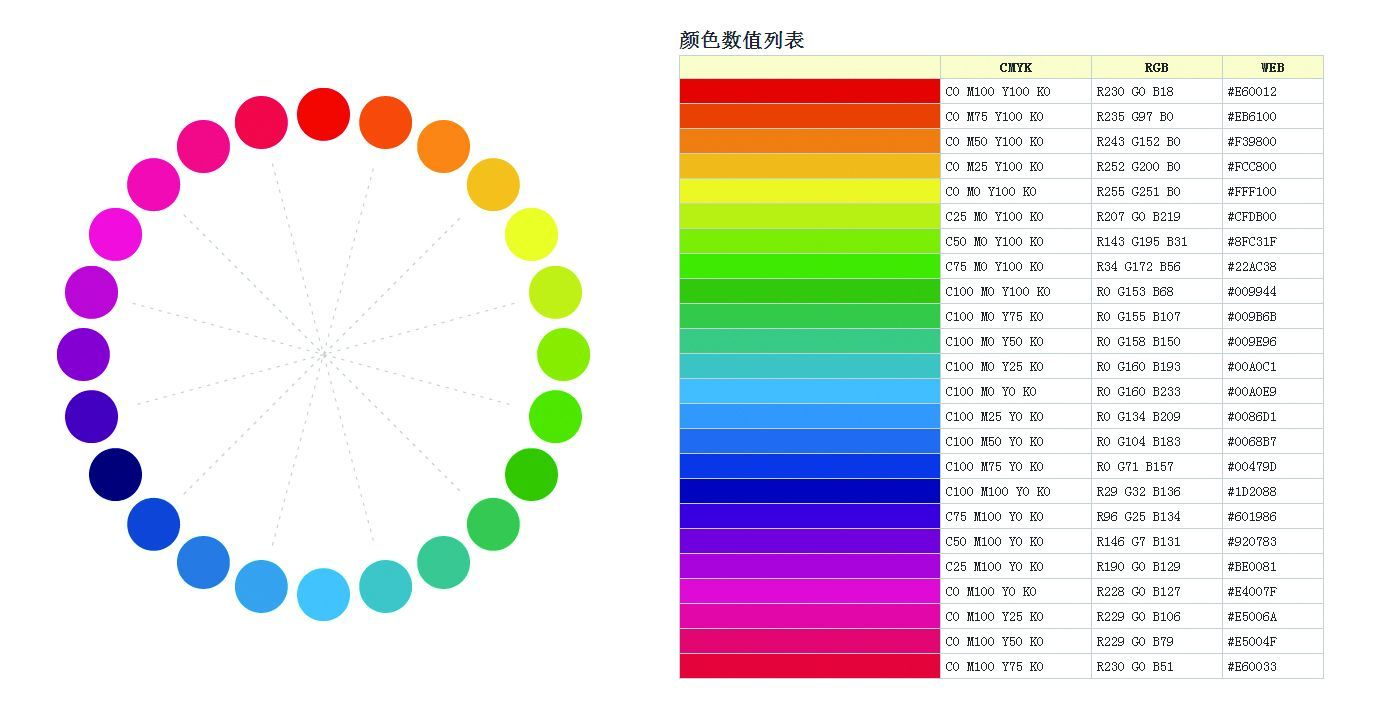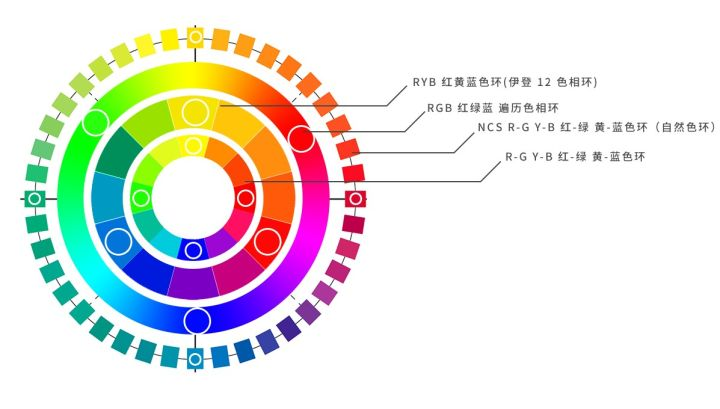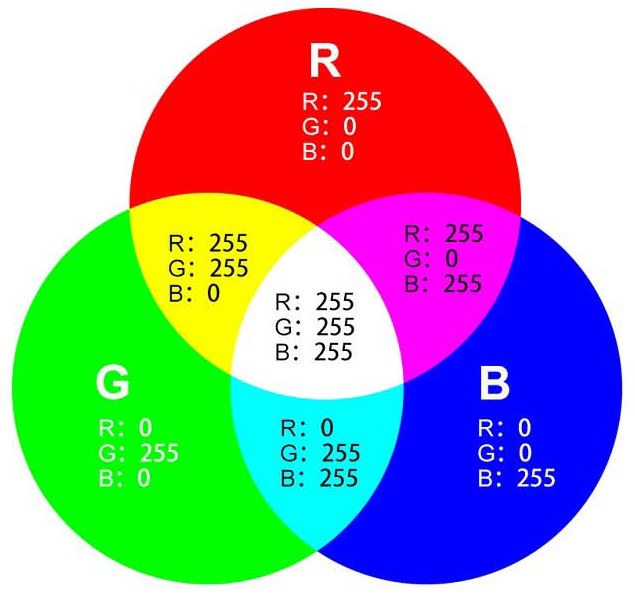# 颜色原理

## 3. 应用

### 3.1 RGB「叠加原理」

• 计算机显示设备、电视机、手机的屏幕基础颜色是黑色。在黑色基础上，如果要想显示颜色，就要采用叠加型原理。叠加原理是指色基的光谱能量分布随能量比例增加，是能量强度的线性叠加。
• RGB 是从发光体原理角度来设计的，R、G、B 依次代表红、绿、蓝三元色，采用了叠加原理。

### 3.2 CMYK「消减原理」

• 印刷、绘画标准中，基础颜色是白色。在白色基础上，如果要想反射颜色，就要采用消减型原理。消减原理是指密度叠加，即染料的谱吸收特性固定不变，在固定的光源下染料密度变化，从而产生反射能量的对数变化，相当于对数叠加再反相。
• CMY 是从物体反射光的角度来设计的，C、M、Y 依次代表青、品红、黄色，由于现实中 CMY 混合的密度无法达到无限大，所以无法出现反射能量为 0 的点，加之 CMY 模式的色彩空间较小，所以为了显示纯黑点，CMYK 模式加上了黑色基色。

### 3.3 RGB 与 CMYK 转换

#### 3.3.1 RGB 到 CMYK

$\begin{array}{rcl} K & = & (2^{0.5p} - 1) - \max{(R,G,B)} \\ C & = & (2^{0.5p} - 1) - R - K \\ Y & = & (2^{0.5p} - 1) - G - K \\ M & = & (2^{0.5p} - 1) - B - K \\ \end{array}$

#### 3.3.2 CMYK 到 RGB

$\begin{array}{rcl} R & = & (2^{0.5p} - 1) - K - C \\ G & = & (2^{0.5p} - 1) - K - Y \\ B & = & (2^{0.5p} - 1) - K - M \\ \end{array}$

## 4. 附录